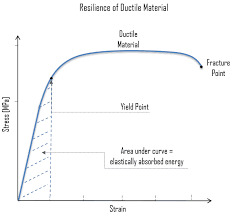## How to Calculate and Solve for Resiliency | Fracture MechanicsThe image above represents resiliency.

To compute for resiliency, two essential parameters are needed and these parameters are Maximum Stress (σmax) and Young’s Modulus of Elasticity (E).

The formula for calculating resiliency:

R = σmax² / 2E

Where:

R = Resiliency
σmax = Maximum Stress
E = Young’s Modulus of Elasticity

Let’s solve an example;
Find the resiliency when the maximum stress is 32 and the young’s modulud of elasticity is 12.

This implies that;

σmax = Maximum Stress = 32
E = Young’s Modulus of Elasticity = 12

R = σmax² / 2E
R = 32² / 2(12)
R = 1024 / 24
R = 42.67

Therefore, the resiliency is 42.67 Pa.

Calculating the Maximum Stress when the Resiliency and the Young’s Modulus of Elasticity is Given.

σmax = R x 2E

Where;

σmax = Maximum Stress
R = Resiliency
E = Young’s Modulus of Elasticity

Let’s solve an example;
Find the maximum stress when the resiliency is 15 and the young’s modulus of elasticity is 3.

This implies that;

R = Resiliency = 15
E = Young’s Modulus of Elasticity = 3

σmax = R x 2E
σmax = 15 x 2 x 3
σmax = 90
σmax = 9.48

Therefore, the maximum stress is 9.48.